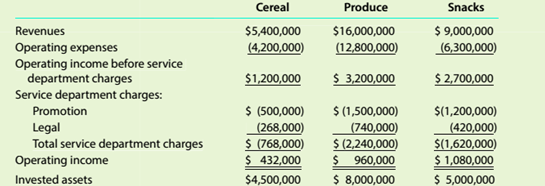Chapter 14, Problem 14.3.1C

Chapter
Section
Textbook Problem

Evaluating divisional performance The three divisions of Dixie Foods are Cereal, Produce, and Snacks. The divisions are structured as investment centers. The following responsihility reports were prepared for the three divisions for the prior year:Which division is making the best use of invested assets and thus should lx- given priority for future capital investments?

To determine

Concept Introduction:

Return on investment (ROI):

Return on investment is a profitability ratio that represents the percentage return on the investment made. It is calculated by dividing the Net Income by the Average total assets. The formulas to calculate the ROI are as follows:

ROI = Operating IncomeAverage total assets

ROI (Expanded)= Operating IncomeSales ×SalesAverage total assets

Or

ROI = Profit Margin Ratio ×Asset Turnover ratio

The Division which is making best use of invested assets

Explanation

Division which is making best use of invested assets is identified as follows:

 Cereal Produce Snacks Operating Income (A) $432,000$ 960,000 \$ 1,080,000

Still sussing out bartleby?

Check out a sample textbook solution.

See a sample solution

The Solution to Your Study Problems

Bartleby provides explanations to thousands of textbook problems written by our experts, many with advanced degrees!

Get Started

Find more solutions based on key concepts